# Dirichlet process mixture models¶

Bayesian mixture models introduced how to infer the posterior of the parameters of a mixture model with a fixed number of components $K$. We can either find $K$ using model selection, i.e. with AIC, BIC, WAIC, etc., or try to automatically infer this number. Nonparametric mixture models do exactly this.

Here we implement a nonparametric Bayesian mixture model using Gibbs sampling. We use a Chinese restaurant process prior and stick-breaking construction to sample from a Dirichlet process (see for instance Nils Hjort's Bayesian Nonparametrics, Peter Orbanz' lecture notes), Kevin Murphy's book and last but not least Herman Kamper's notes.

We'll implement the Gibbs sampler using the CRP ourselves, since (I think) Stan doesn't allow us to do this and then use the stick-breaking construction with Stan. That is technically not possible though, so we use a small hack (a truncated DP).

We need to load some libraries first.

In :
suppressMessages({
library(e1071)
library(mvtnorm)
library(dplyr)
library(ggplot2)
library(MCMCpack)
library(bayesplot)
library(rlang)
library(logisticPCA)
library(abind)
library(rstan)
})

set.seed(23)

In :
rstan_options(auto_write = TRUE)
options(mc.cores = parallel::detectCores())


In Bayesian mixture models we used following hierarchical form to describe a mixture model:

\begin{align*} \boldsymbol \theta_k & \sim \mathcal{G}_0\\ \boldsymbol \pi & \sim \text{Dirichlet}(\boldsymbol \alpha_0)\\ z_i & \sim \text{Discrete}(\boldsymbol \pi)\\ \mathbf{x}_i \mid z_i = k & \sim {P}(\boldsymbol \theta_k) \end{align*}

where $\mathcal{G}_0$ is some base distribution for the model parameters.

The DP on contrast, as any BNP model, puts priors on structures that accomodate infinite sizes. The resulting posteriors give a distribution on structures that grow with new observations. A mixture model using an possibly infinite number of components could look like this:

\begin{align*} \mathcal{G} & \sim \mathcal{DP}(\alpha, \mathcal{G}_0)\\ \boldsymbol \theta_i & \sim \mathcal{G}\\ \mathbf{x}_i& \sim {P}(\boldsymbol \theta_i) \end{align*}

where $\mathcal{G}_0$ is the same base measure as above and $\mathcal{G}$ is a sample from the DP, i.e. also a random measure.

## The Chinese restaurant process¶

One way, and possibly the easiest, to implement a DPMM is using a Chinese restaurant process (CRP) which is a distribution over partitions.

### Data generating process¶

The hierarchical model using a CRP is:

\begin{align*} \boldsymbol \theta_k & \sim \mathcal{G}_0 \\ z_i \mid \mathbf{z}_{1:i-1} & \sim \text{CRP} \\ \mathbf{x}_i & \sim P(\boldsymbol \theta_{z_i}) \end{align*}

where $\text{CRP}$ is a prior on possible infinitely many classes. Specifically the CRP is defined as:

\begin{align*} P(z_i = k \mid \mathbf{z}_{-i}) = \left\{ \begin{array}{ll} \frac{N_k}{N - 1 + \alpha}\\ \frac{\alpha}{N - 1 + \alpha}\\ \end{array} \right. \end{align*}

where $N_k$ is the number of customers at table $k$ and $\alpha$ some hyperparameter.

For the variables of interest, $\boldsymbol \theta_k$ and $\boldsymbol z$ the posterior is:

\begin{align*} P(\boldsymbol \theta, \boldsymbol z \mid \mathbf{X}) \propto P(\mathbf{X} \mid \boldsymbol \theta, \boldsymbol z ) P(\boldsymbol \theta) P ( \boldsymbol z ) \end{align*}

Using a Gibbs sampler, we iterate over the following two steps:

1) sample $z_i \sim P(z_i \mid \mathbf{z}_{-i}, \mathbf{X}, \boldsymbol \theta) \propto P(z_i \mid \mathbf{z}_{-i}) P(\mathbf{x}_i \mid \boldsymbol \theta_{z_i}, \mathbf{X}_{-i}, \mathbf{z})$

2) sample $\boldsymbol \theta_k \sim P(\boldsymbol \theta_k \mid \mathbf{z}, \mathbf{X})$

So we alternate sampling assignments of data to classes and sampling the parameters of the data distribution given the class assignments. The major difference here compared to the finite case is the way of sampling $z_i$ which we do using the CRP in the infinite case. The CRP itself is defined by $P(z_i \mid \mathbf{z}_{-i})$, so replacing this by a usual finite sample would give us a finite mixture. Evaluation of the likelihoods in the first step is fairly straightforward as we will see. Updating the model parameters in the second step is conditional on every class, an by that also not too hard to do.

## Stick-breaking construction¶

With the CRP with put a prior distribution on the possibly infinite number of class assignments. An alternative approach is to use stick-breaking construction. The advantage here is that we could use Stan using a truncated DP, thus we don't need to implement the sampler ourselves.

### Data generating process¶

If we, instead of putting a CRP prior on the latent labels, put a prior on the possibly infinite sequence of mixing weights $\boldsymbol \pi$ we arrive at the stick-breaking construction. The hierarchical model now looks like this:

\begin{align*} \nu_k &\sim \text{Beta}(1, \alpha) \\ \pi_k & = \nu_k \prod_{j=1}^{k-1} (1 - \nu_j) \\ \boldsymbol \theta_k & \sim G_0 \\ \mathbf{x}_i & \sim \sum_k \pi_k P(\boldsymbol \theta_k) \end{align*}

where $N_k$ is the number of customers at table $k$ and $\alpha$ some hyperparameter. The distribution of the mixing weights is sometimes denoted as

$$\boldsymbol \pi \sim \text{GEM}(\alpha)$$

## Gaussian DPMM¶

In the following section, we derive a Gaussian Dirichlet process mixture using the CRP with a Gibbs sampler and the stick-breaking construction using Stan.

### CRP¶

In the Gaussian case the hierarchical model using the CRP has the following form:

\begin{align*} \boldsymbol \Sigma_k & \sim \mathcal{IW}\\ \boldsymbol \mu_k & \sim \mathcal{N}(\boldsymbol \mu_0, \boldsymbol \Sigma_0) \\ z_i \mid z_{1:i-1} & \sim \text{CRP} \\ \mathbf{x}_i & \sim \mathcal{N}(\boldsymbol \mu_{z_i}, \boldsymbol \Sigma_{z_i}) \end{align*}

Let's derive the Gibbs sampler for a infinite Gaussian mixture using the CRP.

First we set data $\mathbf{X}$ some constants. We create a very simple data set to avoid problems with identifiability and label switching. For a treatment of the topic see Michael Betancourt's case study. $n$ is the number of samples, $p$ is the dimensionality of the Gaussian, $\alpha$ is the Dirichlet concentration.

In :
n <- 100
p <- 2
alpha <- .5


Latent class assignments (Z), the current table index and the number of customers per table:

In :
Z <- integer(n)
X <- matrix(0, n, p)
curr.tab <- 0
tables <- c()


Parameters of the Gaussians:

In :
sigma <- .1
mus <- NULL


Then we create a random assignment of customers to tables with probability $P(z_i \mid Z_{-i})$, i.e. we use the CRP to put data into classes. Note that we don't know the number of classes that comes out!

In :
for (i in seq(n))
{
probs <- c(tables / (i - 1 + alpha), alpha / (i - 1 + alpha))
table <- rdiscrete(1, probs)
if (table > curr.tab)
{
curr.tab <- curr.tab + 1
tables <- c(tables, 0)
mu <- mvtnorm::rmvnorm(1, c(0, 0), 10 * diag(p))
mus <- rbind(mus, mu)
}
Z[i] <- table
X[i,] <- mvtnorm::rmvnorm(1, mus[Z[i], ], sigma * diag(p))
tables[table] <-  tables[table] + 1
}


Let's see how many clusters and how many data points per clusters we have.

In :
data.frame(table(Z))%>%
ggplot() +
geom_col(aes(Z, Freq), width=.5) +
theme_minimal()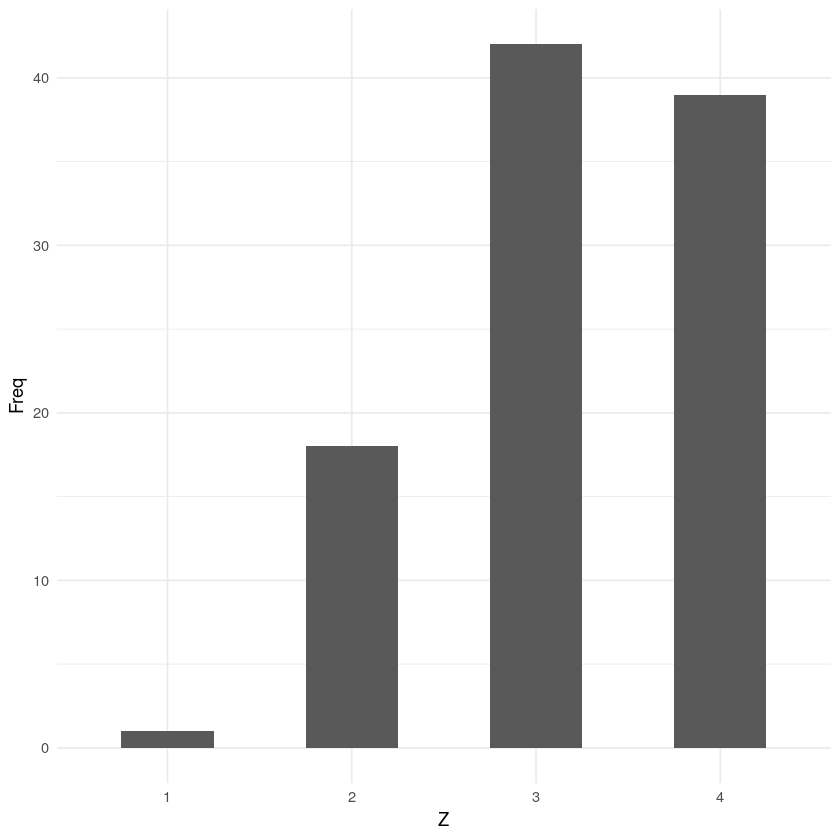In :
data.frame(X=X, Z=as.factor(Z)) %>%
ggplot() +
geom_point(aes(X.1, X.2, col=Z)) +
theme_minimal()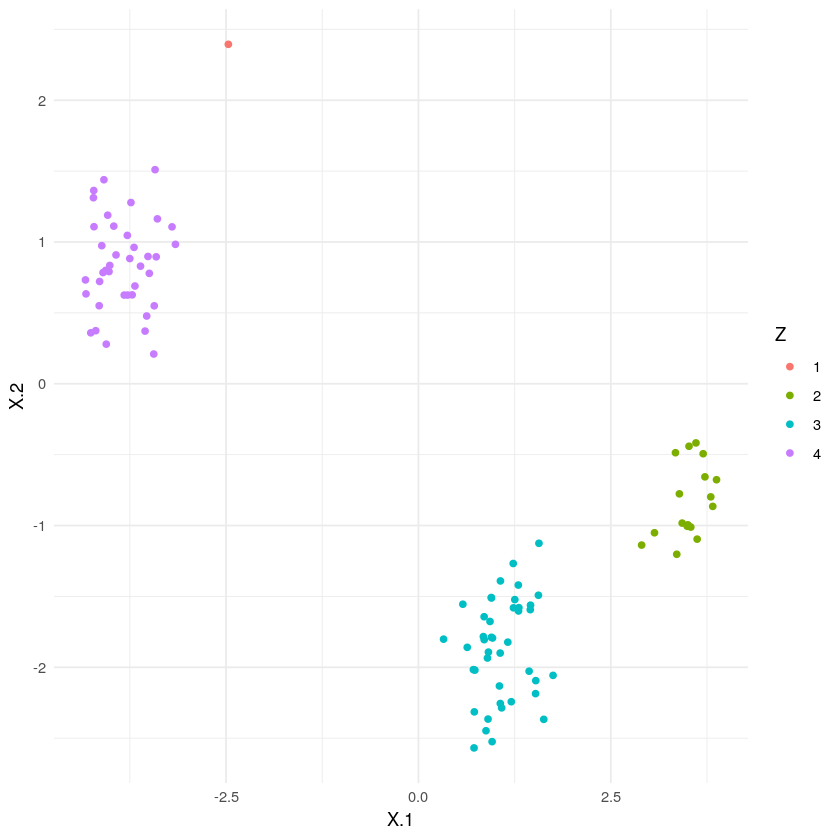#### Posterior inference using Gibbs sampling¶

We randomly initialize the cluster assignments and set all customers to table 1. Hyperparameter $\alpha$ controls the probability of opening a new table.

In :
# initialization of the cluster assignments
K <- 1
zs <- rep(K, n)
alpha <- 5
tables <- n


We assume the covariances to be known.

In :
mu.prior <- matrix(c(0, 0), ncol = 2)
sigma.prior <- diag(p)
q.prior <- solve(sigma.prior)


Base distribution $\mathcal{G}_0$:

In :
sigma0 <- diag(p)
prec0 <- solve(sigma0)
mu0 <- rep(0, p)


To infer the posterior we would use the Gibbs sampler described above. Here, I am only interested in the most likely assignment, i.e. the MAP of $Z$.

In :
for (iter in seq(100))
{
for (i in seq(n))
{
# look at data x_i and romove its statistics from the clustering
zi <- zs[i]
tables[zi] <- tables[zi] - 1
if (tables[zi] == 0) {
K <- K - 1
zs[zs > zi] <- zs[zs > zi] - 1
tables <- tables[-zi]
mu.prior <- mu.prior[-zi, ]
}

# compute posterior probabilitites P(z_i \mid z_-i, ...)
no_i <- seq(n)[-i]
probs <- sapply(seq(K), function(k) {
crp <- sum(zs[no_i] == k) / (n + alpha - 1)
lik <- mvtnorm::dmvnorm(X[i, ], mu.prior[k,], sigma.prior)
crp * lik
})

# compute probability for opening up a new one
crp <- alpha / (n + alpha - 1)
lik <- mvtnorm::dmvnorm(X[i, ], mu0, sigma.prior + sigma0)
probs <- c(probs, crp * lik)
probs <- probs / sum(probs)

# sample new z_i according to the conditional posterior above
z_new <- which.max(probs)
if (z_new > K) {
K <- K + 1
tables <- c(tables, 0)
mu.prior <- rbind(mu.prior, mvtnorm::rmvnorm(1, mu0, sigma0))
}
zs[i] <- z_new
tables[z_new] <- tables[z_new] + 1

# compute conditional posterior P(mu \mid ...)
for(k in seq(K)) {
Xk <- X[zs == k, ,drop=FALSE]
lambda <- solve(q.prior + tables[k] * q.prior)
nominator <- tables[k] * q.prior %*% apply(Xk, 2, mean)
mu.prior[k, ] <- mvtnorm::rmvnorm(1, lambda %*% nominator, lambda)
}
}
}


Let's see if that worked out!

In :
data.frame(X=X, Z=as.factor(zs)) %>%
ggplot() +
geom_point(aes(X.1, X.2, col=Z)) +
theme_minimal()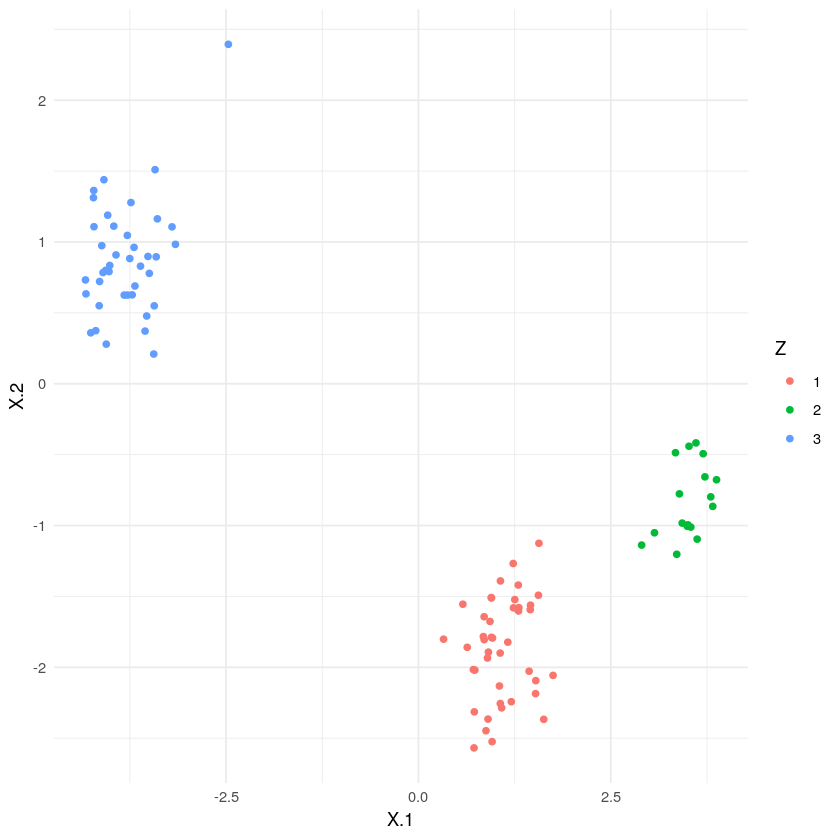Except for the lone guy on top the clustering worked nicely.

### Stick breaking construction¶

In order to make the DPMM with stick-breaking work in Stan, we need to supply a maximum number of clusters $K$ from which we can choose. Setting $K=n$ would mean that we allow that every data point defines its own cluster. For the sake of the exercise I'll set it the maximum number of clusters to $10$. The hyperparameter $\alpha$ parameterizes the Beta-distribution which we use to sample stick lengths. We use the same data we already generated above.

In :
K <- 10
alpha <- 2


The model is a bit more verbose in comparison to the finite case (Bayesian mixture models). We only need to add the stick breaking part in the transformed parameters, the rest stays the same. We again use the LKJ prior for the correlation matrix of the single components and set a fixed prior scale of $1$. In order to get nice, unimodel posteriors, we also introduce an ordering of the mean values.

In :
stan.file <- "_models/dirichlet_process_mixture.stan"

data {
int<lower=0> K;
int<lower=0> n;
int<lower=1> p;
row_vector[p] x[n];
real alpha;
}

parameters {
ordered[p] mu[K];
cholesky_factor_corr[p] L;
real <lower=0, upper=1> nu[K];
}

transformed parameters {
simplex[K] pi;
pi = nu;
for(j in 2:(K-1))
{
pi[j] = nu[j] * (1 - nu[j - 1]) * pi[j - 1] / nu[j - 1];
}

pi[K] = 1 - sum(pi[1:(K - 1)]);
}

model {
real mix[K];

L ~ lkj_corr_cholesky(5);
nu ~ beta(1, alpha);
for (i in 1:K)
{
mu[i] ~ normal(0, 5);
}

for(i in 1:n)
{
for(k in 1:K)
{
mix[k] = log(pi[k]) + multi_normal_cholesky_lpdf(x[i] | mu[k], L);
}
target += log_sum_exp(mix);
}
}

In :
fit <- stan(
stan.file,
data = list(K=K, n=n, x=X, p=p, alpha=alpha),
iter = 10000,
warmup = 1000,
chains = 1
)

SAMPLING FOR MODEL 'dirichlet_process_mixture' NOW (CHAIN 1).
Chain 1:
Chain 1: Gradient evaluation took 0.000499 seconds
Chain 1: 1000 transitions using 10 leapfrog steps per transition would take 4.99 seconds.
Chain 1:
Chain 1:
Chain 1: Iteration:    1 / 10000 [  0%]  (Warmup)
Chain 1: Iteration: 1000 / 10000 [ 10%]  (Warmup)
Chain 1: Iteration: 1001 / 10000 [ 10%]  (Sampling)
Chain 1: Iteration: 2000 / 10000 [ 20%]  (Sampling)
Chain 1: Iteration: 3000 / 10000 [ 30%]  (Sampling)
Chain 1: Iteration: 4000 / 10000 [ 40%]  (Sampling)
Chain 1: Iteration: 5000 / 10000 [ 50%]  (Sampling)
Chain 1: Iteration: 6000 / 10000 [ 60%]  (Sampling)
Chain 1: Iteration: 7000 / 10000 [ 70%]  (Sampling)
Chain 1: Iteration: 8000 / 10000 [ 80%]  (Sampling)
Chain 1: Iteration: 9000 / 10000 [ 90%]  (Sampling)
Chain 1: Iteration: 10000 / 10000 [100%]  (Sampling)
Chain 1:
Chain 1:  Elapsed Time: 60.5386 seconds (Warm-up)
Chain 1:                217.612 seconds (Sampling)
Chain 1:                278.15 seconds (Total)
Chain 1:

Warning message:
“The largest R-hat is NA, indicating chains have not mixed.
Running the chains for more iterations may help. See
http://mc-stan.org/misc/warnings.html#r-hat”
Warning message:
“Bulk Effective Samples Size (ESS) is too low, indicating posterior means and medians may be unreliable.
Running the chains for more iterations may help. See
http://mc-stan.org/misc/warnings.html#bulk-ess”
Warning message:
“Tail Effective Samples Size (ESS) is too low, indicating posterior variances and tail quantiles may be unreliable.
Running the chains for more iterations may help. See
http://mc-stan.org/misc/warnings.html#tail-ess”

In :
fit

Inference for Stan model: dirichlet_process_mixture.
1 chains, each with iter=10000; warmup=1000; thin=1;
post-warmup draws per chain=9000, total post-warmup draws=9000.

mean se_mean   sd    2.5%     25%     50%     75%   97.5% n_eff
mu[1,1]    -3.83    0.00 0.16   -4.14   -3.94   -3.83   -3.72   -3.51  3105
mu[1,2]     0.83    0.00 0.16    0.51    0.72    0.83    0.94    1.15  3370
mu[2,1]    -0.40    0.00 0.04   -0.49   -0.43   -0.40   -0.37   -0.32  4557
mu[2,2]    -0.37    0.00 0.04   -0.45   -0.40   -0.37   -0.34   -0.29  7036
mu[3,1]     1.31    0.00 0.06    1.19    1.27    1.31    1.35    1.43  6064
mu[3,2]     1.36    0.00 0.06    1.24    1.32    1.36    1.40    1.49  7512
mu[4,1]    -2.73    0.05 3.84  -10.62   -5.01   -2.76   -0.23    4.75  4940
mu[4,2]     2.54    0.04 3.90   -4.69    0.08    2.11    4.95   10.98  7536
mu[5,1]    -2.80    0.05 3.94  -11.08   -5.30   -2.72   -0.19    4.70  5537
mu[5,2]     2.70    0.05 4.01   -4.85    0.11    2.38    5.18   11.33  6419
mu[6,1]    -2.89    0.05 4.05  -11.14   -5.49   -2.83   -0.16    4.69  5724
mu[6,2]     2.76    0.04 3.95   -4.70    0.13    2.60    5.30   10.96  9324
mu[7,1]    -2.78    0.06 4.09  -11.14   -5.46   -2.71   -0.11    5.14  4958
mu[7,2]     2.76    0.04 4.14   -4.86   -0.11    2.59    5.49   11.28  8613
mu[8,1]    -2.80    0.05 4.15  -11.29   -5.53   -2.69    0.00    5.22  5825
mu[8,2]     2.81    0.04 4.16   -5.07   -0.04    2.66    5.57   11.48  9361
mu[9,1]    -2.75    0.06 4.13  -11.15   -5.45   -2.67    0.06    5.05  5304
mu[9,2]     2.77    0.04 4.10   -4.91    0.01    2.67    5.48   11.21  8501
mu[10,1]   -2.76    0.06 4.14  -11.29   -5.40   -2.72   -0.06    5.28  5012
mu[10,2]    2.87    0.04 4.11   -4.90    0.09    2.72    5.58   11.08  9137
L[1,1]      1.00     NaN 0.00    1.00    1.00    1.00    1.00    1.00   NaN
L[1,2]      0.00     NaN 0.00    0.00    0.00    0.00    0.00    0.00   NaN
L[2,1]     -0.88    0.00 0.02   -0.91   -0.89   -0.88   -0.87   -0.84  3722
L[2,2]      0.48    0.00 0.03    0.42    0.46    0.48    0.50    0.55  4220
nu       0.38    0.00 0.05    0.29    0.35    0.38    0.42    0.48  6267
nu       0.68    0.00 0.06    0.56    0.64    0.68    0.72    0.79  5209
nu       0.88    0.00 0.08    0.67    0.84    0.90    0.94    0.98  1676
nu       0.35    0.00 0.24    0.01    0.14    0.31    0.52    0.86  4437
nu       0.33    0.00 0.24    0.01    0.13    0.29    0.50    0.84  6578
nu       0.34    0.00 0.24    0.01    0.14    0.30    0.51    0.85  5516
nu       0.33    0.00 0.23    0.01    0.14    0.29    0.50    0.84  6267
nu       0.33    0.00 0.24    0.01    0.13    0.29    0.50    0.84  7093
nu       0.33    0.00 0.24    0.01    0.13    0.29    0.50    0.85  7553
nu      0.33    0.00 0.23    0.01    0.13    0.29    0.50    0.84  6291
pi       0.38    0.00 0.05    0.29    0.35    0.38    0.42    0.48  6267
pi       0.42    0.00 0.05    0.32    0.39    0.42    0.45    0.52  6424
pi       0.17    0.00 0.04    0.11    0.15    0.17    0.20    0.25  6177
pi       0.01    0.00 0.01    0.00    0.00    0.00    0.01    0.04   899
pi       0.00    0.00 0.01    0.00    0.00    0.00    0.01    0.02  3217
pi       0.00    0.00 0.01    0.00    0.00    0.00    0.00    0.02  1264
pi       0.00    0.00 0.00    0.00    0.00    0.00    0.00    0.01  2234
pi       0.00    0.00 0.00    0.00    0.00    0.00    0.00    0.01  4943
pi       0.00    0.00 0.00    0.00    0.00    0.00    0.00    0.01  5497
pi      0.00    0.00 0.00    0.00    0.00    0.00    0.00    0.01  4415
lp__     -406.32    0.09 4.45 -415.89 -409.14 -405.94 -403.12 -398.75  2356
Rhat
mu[1,1]     1
mu[1,2]     1
mu[2,1]     1
mu[2,2]     1
mu[3,1]     1
mu[3,2]     1
mu[4,1]     1
mu[4,2]     1
mu[5,1]     1
mu[5,2]     1
mu[6,1]     1
mu[6,2]     1
mu[7,1]     1
mu[7,2]     1
mu[8,1]     1
mu[8,2]     1
mu[9,1]     1
mu[9,2]     1
mu[10,1]    1
mu[10,2]    1
L[1,1]    NaN
L[1,2]    NaN
L[2,1]      1
L[2,2]      1
nu       1
nu       1
nu       1
nu       1
nu       1
nu       1
nu       1
nu       1
nu       1
nu      1
pi       1
pi       1
pi       1
pi       1
pi       1
pi       1
pi       1
pi       1
pi       1
pi      1
lp__        1

Samples were drawn using NUTS(diag_e) at Thu May 14 15:08:51 2020.
For each parameter, n_eff is a crude measure of effective sample size,
and Rhat is the potential scale reduction factor on split chains (at
convergence, Rhat=1).

High effective sample sizes, no divergent transitions and $\hat{R}$s of one looks good. Our model seems well specified! Let's look at some plots though. First the traces for the means and mixing weights.

In :
posterior <- extract(fit)

In :
options(repr.fig.width=4, repr.plot.height=8)
data.frame(posterior$pi) %>% set_names(paste0("PI_", 1:10)) %>% tidyr::gather(key, value) %>% ggplot(aes(x = value, y = ..density.., fill=key), position="dodge") + facet_grid(key ~ ., scales="free") + geom_histogram(bins=50) + theme_minimal()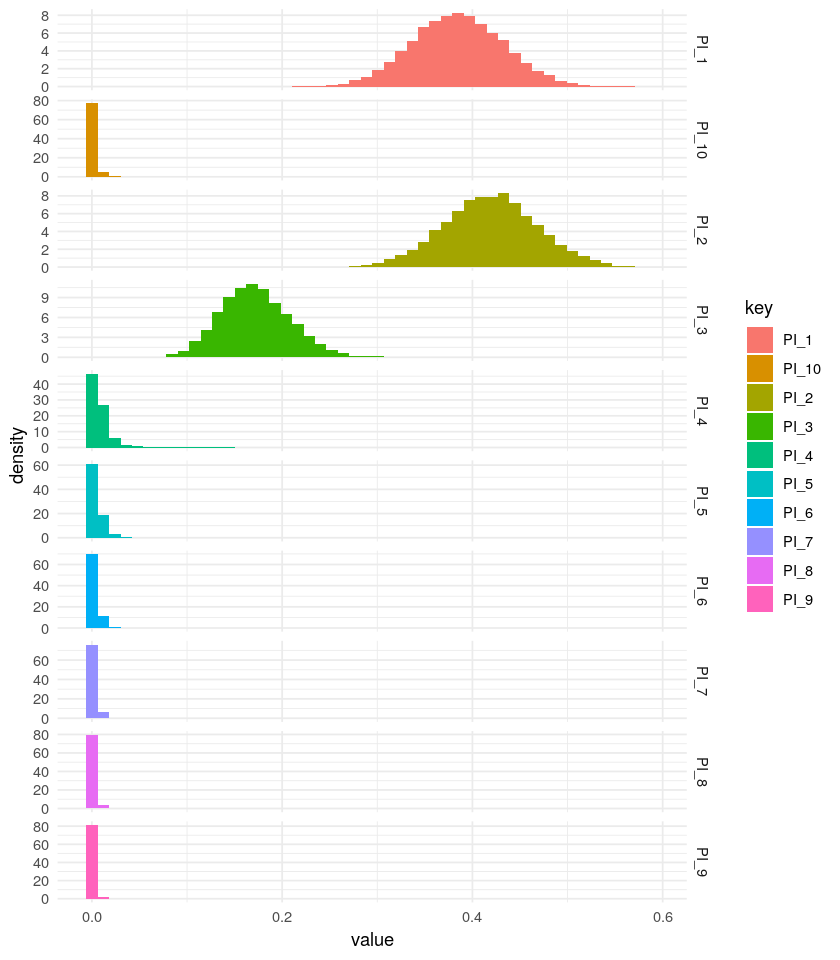From the plot above it looks as if Stan believes it's sufficient to use three components as the means of the mixing weights of the seven other components are fairly low or even zero. However, let's extract all means of the posterior means and assign each data point to a cluster. In : post.mus <- do.call( "rbind", lapply(1:10, function(i) apply(posterior$mu[,i,], 2, mean)))

In :
probs <- purrr::map_dfc(seq(10), function(i) {
mvtnorm::dmvnorm(X, post.mus[i,], diag(2))}) %>%
set_names(paste0("Z", seq(10)))

In :
zs.stan <- apply(probs, 1, which.max)


And the final plot:

In :
options(repr.fig.width=4, repr.plot.height=3)

In :
data.frame(X=X, Z=as.factor(zs.stan)) %>%
ggplot() +
geom_point(aes(X.1, X.2, col=Z)) +
theme_minimal()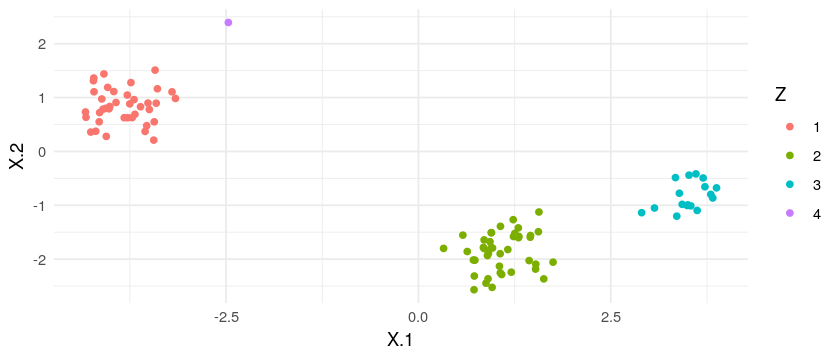Our small hack using Stan and stick-breaking worked even better than our CRP implementation. Here, we managed to give every point its correct label.

## Multivariate Bernoullis¶

Next, we derive a Dirichlet process mixture for a multivariate Bernoulli distribution (or whatever name is more suitable here). We again use the CRP with a Gibbs sampler and the stick-breaking construction using Stan.

### CRP¶

We model every observation $\mathbf{x} \in \{0, 1 \}^p$ as:

\begin{align*} \pi_{{z_i}_j} & \sim \text{Beta}(a, b)\\ z_i \mid z_{1:i-1} & \sim \text{CRP} \\ x_{i, j} \mid z_i & \sim \text{Bernoulli}(\pi_{{z_i}_j}), \end{align*}

with hyperparameters $a$ and $b$. Thus the likelihood for one datum is the product over $p$ independent Bernoullis:

$$P(\mathbf{x} \mid z, \boldsymbol \Pi) = \prod_{j=1}^p \pi_{{z_i}_j}^{x_j} \cdot (1 - \pi_{{z_i}_j})^{(1 - x_j)}$$

First we again generate some data. We sample $100$ points $\mathbf{X}$ from a $p=5$ dimensional mixture of a $k=3$ multivariate Bernoullis and their latent class assignments $\mathbf{z}$ (since we already saw how one can generate data using a CRP).

In :
n <- 200
p <- 3
alpha <- 0.5
k <- 3
Z <- sample(1:k, n, replace = T)
table(Z)

Z
1  2  3
80 70 50 

Then we generate the true success probabilities for every Bernoulli. These are $k \cdot p$ many. We simulate an easy scenario where every dimension has the same probability for every class.

In :
probs.true <- matrix(seq(0.1, 0.9, length.out=k), k, p)
probs.true

 0.1 0.1 0.1 0.5 0.5 0.5 0.9 0.9 0.9

Then we generate the data using these probabilities randomly:

In :
probs.matrix <- probs.true[Z, ]
X  <- (probs.matrix > matrix(runif(n * p), n, p)) * 1L

 0 0 0 1 1 1 1 1 1 0 0 0 0 0 0 1 0 1

Let's have a look at it using $t$-SNE. Note, that since the data are Bernoulli, axes are kinda hard to interpret and we shouldn't get a clear separation of the clusters as in the Gaussian case (the same is true for PCA, too).

In :
log.pca <- logisticPCA(X, k = 2)

In :
g1 <- data.frame(cbind(log.pca$PCs, Z=Z)) %>% ggplot() + geom_point(aes(V1, V2, col=as.factor(Z))) + theme_minimal() g1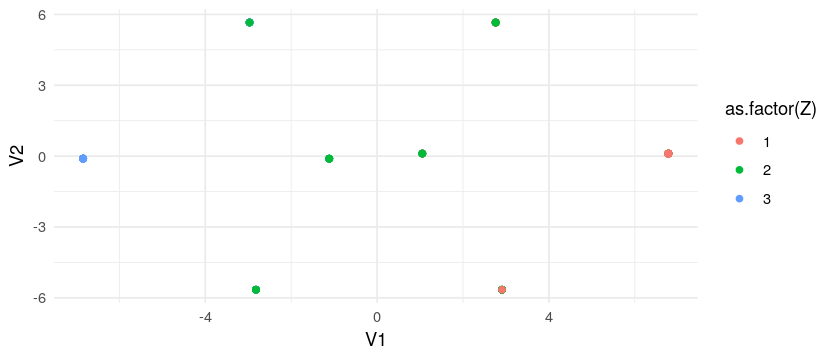We use a concentration of$\alpha=1$for the CRP and$a=b=1$as hyperparameters for the Beta to get a somewhat uniform shape. In : alpha <- 1 a <- b <- 1  As for the Gaussian case, we need to compute the likelihood of$\mathbf{x}_i$evaluated at its cluster$k$. Since we factorize over$p$independent Bernoullis, we need to write down this likelihood manually: In : # likelihood for existing cluster ppd <- function(x, ps) { exp(sum(x * log(ps) + (1 - x) * (1 - log(ps)))) } # likelihood for random new cluster ppde <- function(x) { ps <- rbeta(p, a, b) ppd(x, ps) }  For the sample we start with the following initial parameter settings: one cluster$K=1$, i.e. all latent class assignments are$\mathbf{z} = \{1 \}^n$, and$p$random samples from a Beta for the success probabilities of the Bernoullis determining the cluster. In : K <- 1 zs <- rep(K, n) tables <- n priors <- array(rbeta(p, a, b), dim = c(1, p, 1)) priors  1. 0.813091309275478 2. 0.213314406806603 3. 0.275407212087885 Then we implement the Gibbs sampler (or rather the ECM): In : for (it in seq(100)) { for (i in seq(n)) { # look at data x_i and romove its statistics from the clustering zi <- zs[i] tables[zi] <- tables[zi] - 1 if (tables[zi] == 0) { K <- K - 1 zs[zs > zi] <- zs[zs > zi] - 1 tables <- tables[-zi] priors <- priors[,,-zi,drop=FALSE] } # compute posterior probabilitites P(z_i \mid z_-i, ...) no_i <- seq(n)[-i] probs <- sapply(seq(K), function(k) { crp <- sum(zs[no_i] == k) / (n + alpha - 1) lik <- ppd(X[i, ], priors[,,k]) crp * lik }) # compute probability for opening up a new one crp <- alpha / (n + alpha - 1) lik <- ppde(X[i, ]) probs <- c(probs, crp * lik) probs <- probs / sum(probs) # sample new z_i according to the conditional posterior above z_new <- which.max(probs) if (z_new > K) { K <- K + 1 tables <- c(tables, 0) priors <- abind::abind(priors, array(rbeta(p, a, b), dim=c(1, p, 1))) } zs[i] <- z_new tables[z_new] <- tables[z_new] + 1 # compute conditional posterior P(mu \mid ...) for(k in seq(K)) { Xk <- X[zs == k, ,drop=FALSE] priors[,,k] <- sapply(colSums(Xk), function(i) rbeta(1, i + 1, nrow(Xk) - i + 1)) } } }  Let's compare the inferred assignments with the true ones. In : g2 <- data.frame(cbind(log.pca$PCs, Z=zs)) %>%
ggplot() +
geom_point(aes(V1, V2, col=as.factor(Z))) +
theme_minimal()
cowplot::plot_grid(g1, g2, ncol=2, labels = c("True labels", "Inferred labels"))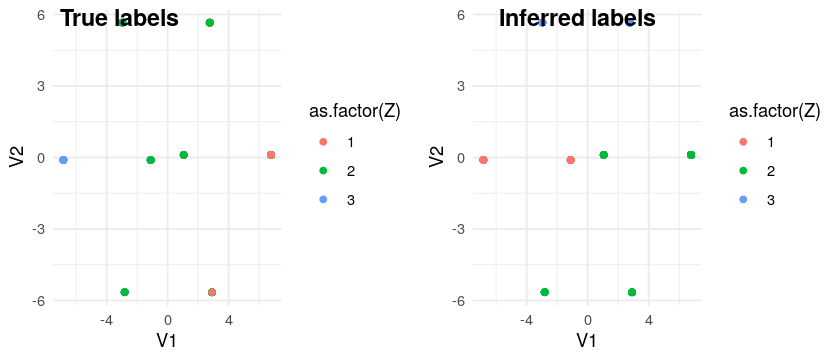### Stick-breaking construction¶

As above, we try to implement the mixture using Stan, too. We use a truncated DP again and set the maximum number of clusters $K =5$, because the model is quite hard to compute and we don't use a lot of data, and the concentration to $\alpha=2$.

In :
K <- 5
alpha <- 2


The respective Stan file is similar to the Gaussian case. There is one more tricky part, though. In the Gaussian case, we needed to order the mean vectors such that we can identifiable posteriors. For the binary case, we in addition need to make sure that the parameters are probabilities, i.e. have a domain from $0$ to $1$. We do that by first declaring a $K \times p$-dimensional parameter rates which we order: ordered[p] rates[K]. Then, in order to make a probability out of it, we apply the inverse logit function for every element: prob = inv_logit(rates). That should do the trick. The complete Stan file is shown below.

In :
stan.file <- "_models/binary_dirichlet_process_mixture.stan"

data {
int<lower=1> K;
int<lower=1> n;
int<lower=1> p;
int<lower=0,upper=1> x[n, p];
real<lower=1> alpha;
}

parameters {
ordered[p] rates[K];
real<lower=0, upper=1> nu[K];
}

transformed parameters {
simplex[K] pi;
vector<lower=0, upper=1>[p] prob[K];

pi = nu;
for(j in 2:(K-1))
{
pi[j] = nu[j] * (1 - nu[j - 1]) * pi[j - 1] / nu[j - 1];
}

pi[K] = 1 - sum(pi[1:(K - 1)]);
for (k in 1:K)
{
for (ps in 1:p)
{
prob[k, ps] = inv_logit(rates[k, ps]);
}
}
}

model {
real mix[K];
nu ~ beta(1, alpha);

for(i in 1:n)
{
for(k in 1:K)
{
mix[k] = log(pi[k]);
for (ps in 1:p)
{
mix[k] += bernoulli_lpmf(x[i, ps] | prob[k, ps]);
}
}
target += log_sum_exp(mix);
}
}

In :
fit <- stan(stan.file, data = list(K=K, n=n, x=matrix(as.integer(X), n, p), p=p, alpha=alpha),
iter = 12000, warmup = 2000, chains = 1, control = list(adapt_delta = 0.99))

SAMPLING FOR MODEL 'binary_dirichlet_process_mixture' NOW (CHAIN 1).
Chain 1:
Chain 1: Gradient evaluation took 0.000188 seconds
Chain 1: 1000 transitions using 10 leapfrog steps per transition would take 1.88 seconds.
Chain 1:
Chain 1:
Chain 1: Iteration:     1 / 12000 [  0%]  (Warmup)
Chain 1: Iteration:  1200 / 12000 [ 10%]  (Warmup)
Chain 1: Iteration:  2001 / 12000 [ 16%]  (Sampling)
Chain 1: Iteration:  3200 / 12000 [ 26%]  (Sampling)
Chain 1: Iteration:  4400 / 12000 [ 36%]  (Sampling)
Chain 1: Iteration:  5600 / 12000 [ 46%]  (Sampling)
Chain 1: Iteration:  6800 / 12000 [ 56%]  (Sampling)
Chain 1: Iteration:  8000 / 12000 [ 66%]  (Sampling)
Chain 1: Iteration:  9200 / 12000 [ 76%]  (Sampling)
Chain 1: Iteration: 10400 / 12000 [ 86%]  (Sampling)
Chain 1: Iteration: 11600 / 12000 [ 96%]  (Sampling)
Chain 1: Iteration: 12000 / 12000 [100%]  (Sampling)
Chain 1:
Chain 1:  Elapsed Time: 66.2135 seconds (Warm-up)
Chain 1:                122.78 seconds (Sampling)
Chain 1:                188.994 seconds (Total)
Chain 1:

Warning message:
“There were 9983 divergent transitions after warmup. Increasing adapt_delta above 0.99 may help. See
http://mc-stan.org/misc/warnings.html#divergent-transitions-after-warmup”
Warning message:
“Examine the pairs() plot to diagnose sampling problems
”
Warning message:
“The largest R-hat is 1.65, indicating chains have not mixed.
Running the chains for more iterations may help. See
http://mc-stan.org/misc/warnings.html#r-hat”
Warning message:
“Bulk Effective Samples Size (ESS) is too low, indicating posterior means and medians may be unreliable.
Running the chains for more iterations may help. See
http://mc-stan.org/misc/warnings.html#bulk-ess”
Warning message:
“Tail Effective Samples Size (ESS) is too low, indicating posterior variances and tail quantiles may be unreliable.
Running the chains for more iterations may help. See
http://mc-stan.org/misc/warnings.html#tail-ess”


That is unpleasant! A lot of divergent transitions and almost all of the transitions exceeded the maximum tree depth (see the Stan manual). The divergent transitions are more severe a problem than the transitions so let's look at some diagnostic plots.

In :
fit

Inference for Stan model: binary_dirichlet_process_mixture.
1 chains, each with iter=12000; warmup=2000; thin=1;
post-warmup draws per chain=10000, total post-warmup draws=10000.

mean se_mean    sd    2.5%     25%     50%     75%   97.5% n_eff
rates[1,1]   -0.64    0.03  0.26   -1.16   -0.80   -0.65   -0.47   -0.14    87
rates[1,2]   -0.41    0.02  0.23   -0.83   -0.58   -0.42   -0.25    0.07   117
rates[1,3]   -0.23    0.02  0.23   -0.65   -0.40   -0.24   -0.09    0.24   152
rates[2,1]   12.70    1.01  7.79    1.61    6.04   11.72   18.61   28.62    59
rates[2,2]   20.75    0.85  8.14    5.36   14.31   21.67   27.35   34.32    93
rates[2,3]   28.86    0.45  6.52   12.04   25.23   30.40   34.06   36.53   214
rates[3,1]    3.78    3.48  7.93   -8.72   -2.28    1.90    9.41   20.44     5
rates[3,2]   14.88    2.77  9.77   -3.56    7.66   15.15   22.24   31.82    12
rates[3,3]   25.54    0.58  7.90    7.11   20.68   26.95   31.96   36.15   186
rates[4,1] -222.62   47.84 89.42 -335.27 -276.21 -250.72 -176.69  -19.72     3
rates[4,2] -167.47   34.49 93.12 -326.09 -237.05 -176.02  -79.22    5.20     7
rates[4,3]  -77.03   10.41 73.94 -242.33 -126.42  -62.17  -17.50   29.94    50
rates[5,1] -160.76   26.06 49.66 -228.53 -196.26 -171.16 -132.23  -43.24     4
rates[5,2] -107.96   16.04 49.07 -192.03 -145.18 -111.40  -70.67  -16.35     9
rates[5,3]  -51.93    5.19 41.28 -144.32  -79.75  -42.17  -18.95   -1.03    63
nu         0.65    0.01  0.06    0.53    0.61    0.65    0.68    0.76   104
nu         0.41    0.03  0.16    0.06    0.32    0.43    0.52    0.69    22
nu         0.20    0.03  0.17    0.00    0.06    0.15    0.29    0.60    25
nu         0.31    0.02  0.23    0.02    0.12    0.27    0.45    0.87   140
nu         0.32    0.03  0.24    0.01    0.12    0.28    0.49    0.85    83
pi         0.65    0.01  0.06    0.53    0.61    0.65    0.68    0.76   104
pi         0.14    0.01  0.05    0.02    0.11    0.15    0.18    0.24    19
pi         0.04    0.01  0.04    0.00    0.01    0.03    0.06    0.16    18
pi         0.05    0.00  0.04    0.00    0.02    0.04    0.07    0.17   173
pi         0.11    0.01  0.06    0.02    0.07    0.11    0.15    0.24   112
prob[1,1]     0.35    0.01  0.06    0.24    0.31    0.34    0.38    0.47    87
prob[1,2]     0.40    0.01  0.06    0.30    0.36    0.40    0.44    0.52   117
prob[1,3]     0.44    0.00  0.06    0.34    0.40    0.44    0.48    0.56   151
prob[2,1]     0.98    0.00  0.07    0.83    1.00    1.00    1.00    1.00   307
prob[2,2]     1.00    0.00  0.03    1.00    1.00    1.00    1.00    1.00   846
prob[2,3]     1.00    0.00  0.02    1.00    1.00    1.00    1.00    1.00  1034
prob[3,1]     0.62    0.16  0.42    0.00    0.09    0.87    1.00    1.00     7
prob[3,2]     0.92    0.04  0.23    0.03    1.00    1.00    1.00    1.00    41
prob[3,3]     1.00    0.00  0.04    1.00    1.00    1.00    1.00    1.00   869
prob[4,1]     0.00    0.00  0.01    0.00    0.00    0.00    0.00    0.00  1081
prob[4,2]     0.03    0.02  0.16    0.00    0.00    0.00    0.00    0.99    42
prob[4,3]     0.12    0.03  0.32    0.00    0.00    0.00    0.00    1.00    84
prob[5,1]     0.00    0.00  0.00    0.00    0.00    0.00    0.00    0.00   658
prob[5,2]     0.00    0.00  0.05    0.00    0.00    0.00    0.00    0.00   930
prob[5,3]     0.03    0.01  0.15    0.00    0.00    0.00    0.00    0.26   355
lp__       -375.30    1.02  4.09 -384.23 -377.75 -374.96 -372.47 -368.25    16
Rhat
rates[1,1] 1.02
rates[1,2] 1.00
rates[1,3] 1.00
rates[2,1] 1.02
rates[2,2] 1.00
rates[2,3] 1.01
rates[3,1] 1.21
rates[3,2] 1.07
rates[3,3] 1.01
rates[4,1] 1.63
rates[4,2] 1.28
rates[4,3] 1.06
rates[5,1] 1.42
rates[5,2] 1.09
rates[5,3] 1.04
nu      1.02
nu      1.01
nu      1.03
nu      1.00
nu      1.00
pi      1.02
pi      1.03
pi      1.02
pi      1.00
pi      1.00
prob[1,1]  1.02
prob[1,2]  1.00
prob[1,3]  1.00
prob[2,1]  1.01
prob[2,2]  1.00
prob[2,3]  1.00
prob[3,1]  1.05
prob[3,2]  1.01
prob[3,3]  1.00
prob[4,1]  1.00
prob[4,2]  1.03
prob[4,3]  1.02
prob[5,1]  1.00
prob[5,2]  1.00
prob[5,3]  1.00
lp__       1.11

Samples were drawn using NUTS(diag_e) at Thu May 14 15:12:05 2020.
For each parameter, n_eff is a crude measure of effective sample size,
and Rhat is the potential scale reduction factor on split chains (at
convergence, Rhat=1).
In :
posterior_cp_pi <- as.array(fit, pars = c("pi"))
posterior_cp_prob <- as.array(fit, pars = c("prob"))
np_cp <- nuts_params(fit)

In :
mcmc_trace(posterior_cp_pi,np = np_cp)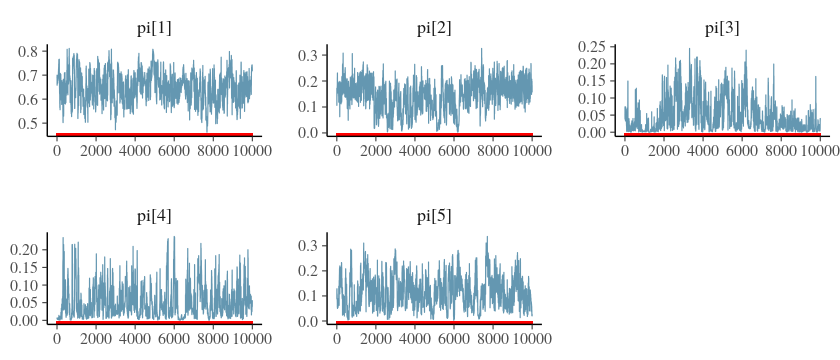Overall the transitions divergence at allmost all points, the traces are not consequently not very nice either. Let's also look at the effective sample size.

In :
ratios_cp <- neff_ratio(fit)
mcmc_neff(ratios_cp)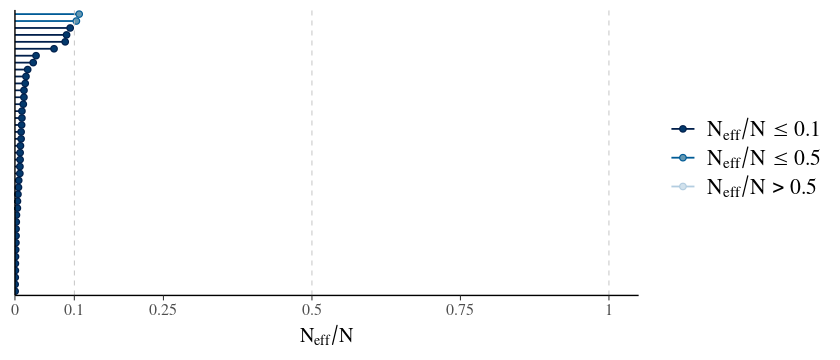Most of the effective sample sizes are extremely low. Before we go on, we should try to change our model, since often badly setup models cause unpleasant posterior analytics. In the case that Stan doesn't like non-parametrics (the truncated stick-breaking), we could test the same model and fix the number of clusters.

In :
stan.file <- "_models/binary_mixture.stan"

data {
int<lower=1> K;
int<lower=1> n;
int<lower=1> p;
int<lower=0,upper=1> x[n, p];
vector<lower=0>[K] alpha;
}

parameters {
ordered[p] rates[K];
simplex[K] pi;
}

transformed parameters {
vector<lower=0, upper=1>[p] prob[K];

for (k in 1:K)
{
for (ps in 1:p)
{
prob[k, ps] = inv_logit(rates[k, ps]);
}
}
}

model {
real mix[K];
pi ~ dirichlet(alpha);

for(i in 1:n)
{
for(k in 1:K)
{
mix[k] = log(pi[k]);
for (ps in 1:p)
{
mix[k] += bernoulli_lpmf(x[i, ps] | prob[k, ps]);
}
}
target += log_sum_exp(mix);
}
}

In :
fit_fixed_K <- stan(stan.file, data = list(K=K, n=n, x=X, p=p, alpha=rep(1.0, K)),
iter = 10000, warmup = 1000, chains = 1, control = list(adapt_delta = 0.99))

SAMPLING FOR MODEL 'binary_mixture' NOW (CHAIN 1).
Chain 1:
Chain 1: Gradient evaluation took 0.000173 seconds
Chain 1: 1000 transitions using 10 leapfrog steps per transition would take 1.73 seconds.
Chain 1:
Chain 1:
Chain 1: Iteration:    1 / 10000 [  0%]  (Warmup)
Chain 1: Iteration: 1000 / 10000 [ 10%]  (Warmup)
Chain 1: Iteration: 1001 / 10000 [ 10%]  (Sampling)
Chain 1: Iteration: 2000 / 10000 [ 20%]  (Sampling)
Chain 1: Iteration: 3000 / 10000 [ 30%]  (Sampling)
Chain 1: Iteration: 4000 / 10000 [ 40%]  (Sampling)
Chain 1: Iteration: 5000 / 10000 [ 50%]  (Sampling)
Chain 1: Iteration: 6000 / 10000 [ 60%]  (Sampling)
Chain 1: Iteration: 7000 / 10000 [ 70%]  (Sampling)
Chain 1: Iteration: 8000 / 10000 [ 80%]  (Sampling)
Chain 1: Iteration: 9000 / 10000 [ 90%]  (Sampling)
Chain 1: Iteration: 10000 / 10000 [100%]  (Sampling)
Chain 1:
Chain 1:  Elapsed Time: 41.0239 seconds (Warm-up)
Chain 1:                631.988 seconds (Sampling)
Chain 1:                673.012 seconds (Total)
Chain 1:

Warning message:
“There were 7984 divergent transitions after warmup. Increasing adapt_delta above 0.99 may help. See
http://mc-stan.org/misc/warnings.html#divergent-transitions-after-warmup”
Warning message:
“There were 1016 transitions after warmup that exceeded the maximum treedepth. Increase max_treedepth above 10. See
http://mc-stan.org/misc/warnings.html#maximum-treedepth-exceeded”
Warning message:
“Examine the pairs() plot to diagnose sampling problems
”
Warning message:
“The largest R-hat is 1.86, indicating chains have not mixed.
Running the chains for more iterations may help. See
http://mc-stan.org/misc/warnings.html#r-hat”
Warning message:
“Bulk Effective Samples Size (ESS) is too low, indicating posterior means and medians may be unreliable.
Running the chains for more iterations may help. See
http://mc-stan.org/misc/warnings.html#bulk-ess”
Warning message:
“Tail Effective Samples Size (ESS) is too low, indicating posterior variances and tail quantiles may be unreliable.
Running the chains for more iterations may help. See
http://mc-stan.org/misc/warnings.html#tail-ess”

In :
fit_fixed_K

Inference for Stan model: binary_mixture.
1 chains, each with iter=10000; warmup=1000; thin=1;
post-warmup draws per chain=9000, total post-warmup draws=9000.

mean se_mean    sd    2.5%     25%     50%     75%   97.5% n_eff
rates[1,1]   -1.64   14.04 24.98  -53.99  -22.00    8.87   16.82   27.92     3
rates[1,2]    7.66   10.86 21.37  -43.06   -6.90   15.06   23.98   31.93     4
rates[1,3]   19.88    8.19 18.15  -26.22   10.65   28.15   33.24   36.41     5
rates[2,1]    9.73    5.31 10.99   -6.02    0.81    6.57   17.38   30.25     4
rates[2,2]   17.45    3.72 10.90   -3.18    9.22   17.81   27.20   33.57     9
rates[2,3]   27.28    1.49  8.67    4.01   23.07   30.20   34.00   36.44    34
rates[3,1]  -41.63   12.55 22.63  -76.17  -63.75  -39.36  -20.52   -6.37     3
rates[3,2]  -22.90    6.88 20.17  -65.04  -35.33  -22.59   -9.05   16.20     9
rates[3,3]   -9.80    6.82 18.62  -44.46  -21.19  -10.60   -3.20   32.48     7
rates[4,1]   -0.60    0.05  0.28   -1.12   -0.79   -0.60   -0.40   -0.05    28
rates[4,2]   -0.38    0.06  0.28   -0.88   -0.57   -0.39   -0.21    0.28    25
rates[4,3]   -0.21    0.07  0.30   -0.76   -0.40   -0.24   -0.04    0.48    19
rates[5,1]  -34.58    3.56  6.33  -44.65  -39.78  -34.94  -30.23  -21.50     3
rates[5,2]  -17.13    3.75 15.92  -43.40  -28.38  -18.44   -7.23   17.00    18
rates[5,3]    7.02    3.27 18.46  -27.96   -7.21    6.61   23.90   35.42    32
pi         0.11    0.03  0.06    0.02    0.04    0.12    0.16    0.21     4
pi         0.08    0.04  0.07    0.00    0.02    0.05    0.15    0.22     4
pi         0.13    0.03  0.08    0.00    0.06    0.14    0.20    0.29    11
pi         0.65    0.00  0.05    0.54    0.61    0.64    0.68    0.76   123
pi         0.03    0.03  0.06    0.00    0.00    0.00    0.01    0.18     5
prob[1,1]     0.65    0.27  0.47    0.00    0.00    1.00    1.00    1.00     3
prob[1,2]     0.70    0.23  0.45    0.00    0.00    1.00    1.00    1.00     4
prob[1,3]     0.79    0.16  0.40    0.00    1.00    1.00    1.00    1.00     6
prob[2,1]     0.80    0.07  0.32    0.00    0.69    1.00    1.00    1.00    19
prob[2,2]     0.93    0.04  0.22    0.04    1.00    1.00    1.00    1.00    30
prob[2,3]     1.00    0.00  0.04    0.98    1.00    1.00    1.00    1.00   401
prob[3,1]     0.00    0.00  0.02    0.00    0.00    0.00    0.00    0.00   240
prob[3,2]     0.13    0.11  0.33    0.00    0.00    0.00    0.00    1.00     8
prob[3,3]     0.19    0.16  0.38    0.00    0.00    0.00    0.04    1.00     6
prob[4,1]     0.36    0.01  0.06    0.25    0.31    0.35    0.40    0.49    28
prob[4,2]     0.41    0.01  0.07    0.29    0.36    0.40    0.45    0.57    25
prob[4,3]     0.45    0.02  0.07    0.32    0.40    0.44    0.49    0.62    19
prob[5,1]     0.00    0.00  0.00    0.00    0.00    0.00    0.00    0.00   136
prob[5,2]     0.14    0.07  0.34    0.00    0.00    0.00    0.00    1.00    25
prob[5,3]     0.58    0.13  0.48    0.00    0.00    1.00    1.00    1.00    15
lp__       -383.51    0.92  4.06 -392.96 -385.87 -383.01 -380.60 -377.05    20
Rhat
rates[1,1] 1.71
rates[1,2] 1.56
rates[1,3] 1.43
rates[2,1] 1.30
rates[2,2] 1.08
rates[2,3] 1.04
rates[3,1] 2.24
rates[3,2] 1.44
rates[3,3] 1.32
rates[4,1] 1.00
rates[4,2] 1.09
rates[4,3] 1.12
rates[5,1] 1.89
rates[5,2] 1.06
rates[5,3] 1.01
pi      1.70
pi      1.54
pi      1.10
pi      1.00
pi      1.31
prob[1,1]  1.83
prob[1,2]  1.59
prob[1,3]  1.32
prob[2,1]  1.01
prob[2,2]  1.00
prob[2,3]  1.00
prob[3,1]  1.01
prob[3,2]  1.15
prob[3,3]  1.23
prob[4,1]  1.00
prob[4,2]  1.09
prob[4,3]  1.12
prob[5,1]  1.01
prob[5,2]  1.00
prob[5,3]  1.05
lp__       1.00

Samples were drawn using NUTS(diag_e) at Thu May 14 15:23:21 2020.
For each parameter, n_eff is a crude measure of effective sample size,
and Rhat is the potential scale reduction factor on split chains (at
convergence, Rhat=1).

That didn't seem to help at all. So the problem is apparently stemming from the multivariate Bernoulli, which makes somewhat sense, since binary data isn't very talkative in the first place, so making informed inferences on these data sets is difficult. For binary data, collapsing the parameters and only using latent class assignments seems to be preferrable. Thus, the next notebook will be on efficient inference of class assignments using particle MCMC.